# Brownian Bridge and Discrete Random Variables

The new Heston discretisation scheme I wrote about a few weeks ago makes use a discrete random variable matching the first five moments of the normal distribution instead of the usual normally distributed random variable, computed via the inverse cumulative distribution function. Their discrete random variable is: $$\xi = \sqrt{1-\frac{\sqrt{6}}{3}} \quad \text{ if } U_1 < 3\,,$$ $$\xi =-\sqrt{1-\frac{\sqrt{6}}{3}} \quad \text{ if } U_1 > 4\,,$$ $$\xi = \sqrt{1+\sqrt{6}} \quad \text{ if } U_1 = 3\,,$$ $$\xi = -\sqrt{1+\sqrt{6}} \quad \text{ if } U_1 = 4\,,$$ with $$U_1 \in \{0,1,…,7\}$$

The advantage of the discrete variable is that it is much faster to generate. But there are some interesting side-effects. The first clue I found is a loss of accuracy on forward-start vanilla options.

By accident, I found a much more interesting side-effect: you can not use the Brownian-Bridge variance reduction on the discrete random variable. This is very well illustrated by the case of a digital option in the Black model, for example with volatility 10% and a 3 months maturity, zero interest rate and dividends. For the following graph, I use 16000 Sobol paths composed of 100 time-steps.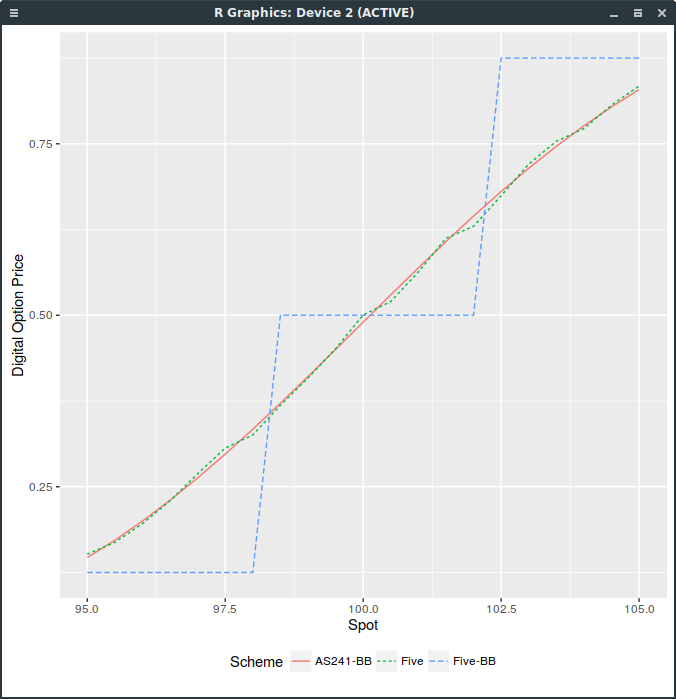Digital Call price with different random variables.

The “-BB” suffix stands for the Brownian-Bridge path construction, “Five” for five moments discrete variable and “AS241” for the inverse cumulative distribution function (continuous approach). As you can see, the price is discrete, and follows directly from the discrete distribution. The use of any random number generator with a large enough number of paths would lead to the same conclusion.

This is because with the Brownian-Bridge technique, the last point in the path, corresponding to the maturity, is sampled first, and the other path points are then completed inside from the first and last points. But the digital option depends only on the value of the path at maturity, that is, on this last point. As this point corresponds follows our discrete distribution, the price of the digital option is a step function.

In contrast, for the incremental path construction, each point is computed from the previous point. The last point will thus include the variation of all points in the path, which will be very close to normal, even with a discrete distribution per point.

The take-out to price more exotic derivatives (including forward-start options) with discrete random variables and the incremental path construction, is that several intermediate time-steps (between payoff observations) are a must-have with discrete random variables, however small is the original time-step size.

Furthermore, one can notice the discrete staircase even with a relavely small time-step for example of 132 (meaning 8 intermediate time-steps in our digital option example). I suppose this is a direct consequence of the digital payoff discontinuity. In Talay “Efficient numerical schemes for the approximation of expectations of functionals of the solution of a SDE, and applications” (which you can read by adding .sci-hub.cc to the URL host name), second order convergence is proven only if the payoff function and its derivatives up to order 6 are continuous. There is something natural that a discrete random variable imposes continuity conditions on the payoff, not necessary with a continuous, smooth random variable: either the payoff or the distribution needs to be smooth.

# Samsung Wireless Printer under Fedora 25

This is a note for those who want to setup a Samsung wireless printer under Linux. It is quite simple, this forum post helped me, the actual useful steps on Fedora 25 are:

• download tar.gz linux driver from Samsung website. As root, unpack & install:

tar xvzf SamsungPrinterInstaller.tar.gz
cd uld
./install.sh

• in the printer menu, lookup for the wireless key (8 digits),

• connect to the printer Wifi network with a computer using the wireless key,

• enter the network gateway IP, this is typically http://192.168.3.1,

• click on Login with user “admin” and password “sec00000”,

• open the network wifi settings and select “Easy Wi-Fi Settings”,

• connect back to your wireless network,

• add the network printer via the printer settings wizard, or alternatively through CUPS interface http://localhost:631.

That’s it. Printer and scanner will then work on your local Wi-Fi network.

# A new scheme for Heston - Part 2

A couple weeks ago, I wrote about a new Heston discretisation scheme which was at least as accurate as Andersen QE scheme and faster, called DVSS2.

It turns out that it does not behave very well on the following Vanilla forward start option example (which is quite benign). The Heston parameters comes from a calibration to the market and are

$$v_0= 0.0718, \kappa= 1.542, \theta= 0.0762, \sigma= 0.582, \rho= -0.352$$

with a maturity of one year.

On a standard vanilla option, DVSS2 behaves as advertised in the paper but not on a forward-start option with forward start date at $$T_1=\frac{7}{8}$$ (relatively close to the maturity). A forward start call option will pay $$\max(S(T)-k S(T_1),0)$$. This is particularly visible on the following graph of the price against the time-step size (1,12,14,18,116,132), for strikes 100% and 140% (it works well for strike=70%) where 32 time-steps are necessary.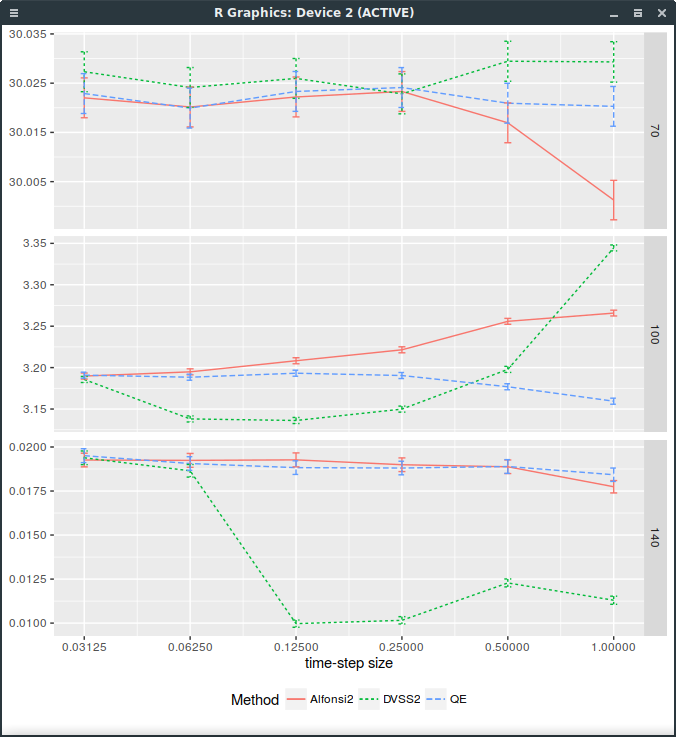Forward start Call price with different discretization schemes.

It would appear that the forward dynamic is sometimes poorly captured by the DVSS2 scheme. This makes DVSS2 not competitive in practice compared to Andersen’s QE or even Alfonsi as it can not be trusted for a time step larger than 132. Note that I insert an extra step at 78 for time step sizes greater or equal than 14: a time-step size of 1 corresponds in reality to two time-steps respectively of size 78 and 18.

The error is actually because the log-asset process is sampled using a discrete random variables that matches the first 5 moments of the normal distribution. The so-called step 5 of the algorithm specifies: $$\hat{X} := \bar{x} + \xi \sqrt{\frac{1}{2}(\bar{y}+\hat{Y})h}$$ The notation is quite specific to the paper, what you need to know is that $$\hat{X}$$ corresponds to the log-asset process while $$\hat{Y}$$ corresponds to the stochastic volatility process and $$\xi$$ is the infamous discrete random variable.

In reality, there is no good reason to use a discrete random variable beside lowering the computational cost. And it is obviously detrimental in the limit case where the volatility is deterministic (Black-Scholes case) as then the log-process will only match the first 5 moments of the normal distribution, while it should be exactly normal.

Replacing $$\xi$$ by a standard normally distributed random variable is enough to fix DVSS2. Note that it could also be discretized like the QE scheme, using a Broadie-Kaya interpolation scheme.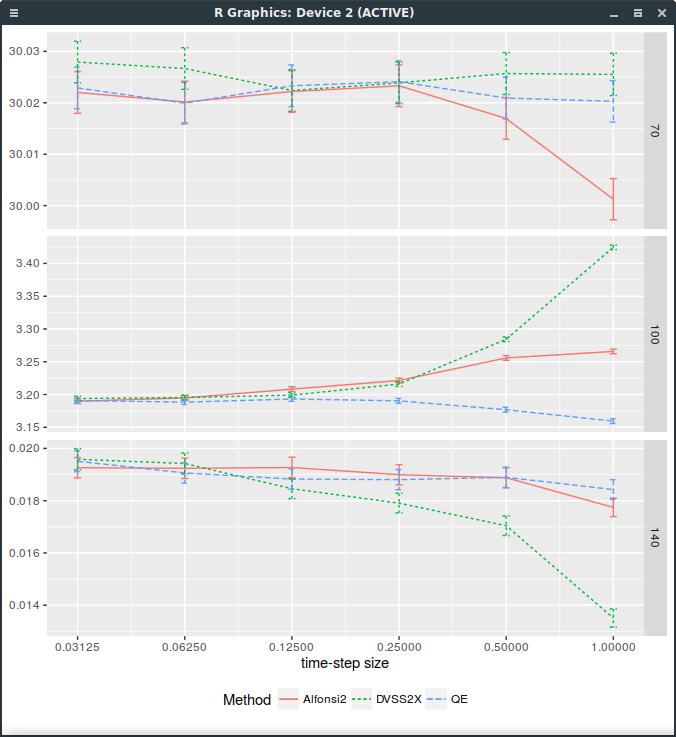Forward start Call price with different discretization schemes. DVSS2X denotes here the scheme with continuous normal random variable.

The problem is that then, it is not faster than QE anymore. So it is not clear why it would be preferable.

### A discrete distribution matching the first 9 moments of the normal distribution

I then tried to see if matching more moments with a discrete distribution would help. More than 8 bits are available when generating a uniform random double precision number (as it is represented by 53 bits). The game is then to find nodes so that the distribution with discrete probabilities in i/256 with some interger i match the moments of the normal distribution. It is a non linear problem unfortunately, but I found a solution for the probabilities

1/256, 1/256, 32/256, 94/256, 94/256, 32/256, 1/256, 1/256
The nodes are:
-3.1348958252117543, -2.6510063991157273, -1.6397086267587215, -0.5168230803049496, 0.5168230803049496, 1.6397086267587215, 2.6510063991157273, 3.1348958252117543
The probabilities approximate those given by the ideal solution on 8 nodes, that is, the weights of the Gauss-Hermite polynomial (see Alan Miller Discrete Approximations of Probability Distributions).

While this helps a bit for small steps as shown on the following graph, it is far from good: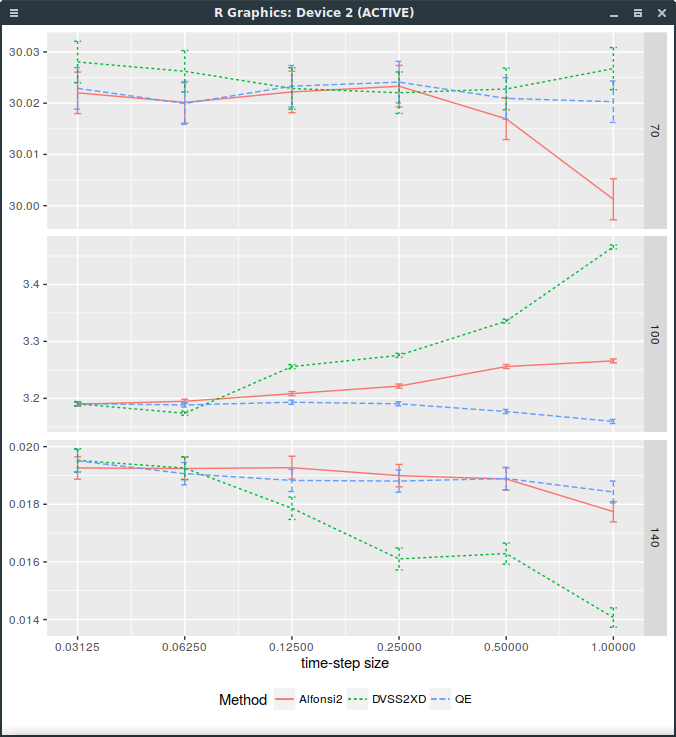Forward start Call price with different discretization schemes. DVSS2X denotes here the scheme with discrete random variable matching the first 9 moments of the normal distribution.

# Power management and XFCE under Fedora 25

The gnome shell has been crashing on me more regularly lately. XFCE is a good and fast more tradional desktop, but, from past experiences, it does not play well with power management if you only install it via

dnf install @xfce-desktop-environment


My typical experience is a black screen after resuming from suspend (sometimes, not always), or hibernate (always) and most of the time I end up just rebooting. It turns out this is all caused by the interaction between the gdm login daemon and xfce. Moving to the lightdm login daemon instead fixes those issues for Fedora 25:

systemctl disable gdm
systemctl enable lightdm
systemctl stop gdm
systemctl start lightdm


# Equivalence between floating-strike and fixed-strike Asian options

Many papers present formulae to price Asian options in the Black-Scholes world only for the fixed strike Asian case, that is a contract that pays $$\max(A-K,0)$$ at maturity $$T$$ where $$A = \sum_{i=0}^{n-1} w_i S(t_i)$$ is the Asian average.

More generally, this can be seen as the payoff of a Basket option where the underlyings are just the same asset but at different times. And any Basket option formula can actually be used to price fixed-strike Asian options by letting the correlation correspond to the correlation between the asset at the averaging times and the variances correspond to the variance at each averaging time. The basket approach allows then naturally for a term-structure of rates, dividends and volatilities.

In order to price floating-strike Asian options paying $$\max(S-kA,0)$$ at maturity $$T$$ where $$k$$ is a percent strike, we can use a change of measure to express it as the price of a fixed-strike Asian. This equivalence is presented in several paperS: it is somewhat hidden in Andreasen “The pricing of discretely sampled Asian and lookback options: a change of numeraire approach”, more explicit in Eberlein and Papapantoleon “Equivalence of floating and fixed strike Asian and lookback options” or Henderson et al. “Bounds for in-progress floating-strike Asian options using symmetry”. None of those really consider the simple case of Black-Scholes with a term-structure of rates, dividends and volatilities.

We have: $$V_{\textsf{floating}}(\eta,S_0,k,\bar{\sigma}_i^2 t_i, C(0,t_i), B(0,T)) =\ \quad k V_{\textsf{fixed}}\left(-\eta,S_0, \frac{S_0}{k},\bar{\sigma}^2(T) T-\bar{\sigma}_i^2 t_i,\frac{C(0,t_i)}{C(0,T)},B(0,T)C(0,T) \right)$$ where $$\eta = \pm 1$$ for a call (respectively a put), $$\bar{\sigma}_i$$ are the Vanilla options implied volatilities at the averaging times $$t_i$$, $$C(0,t_i)$$ are the capitalization factors, and $$B(0,T)$$ is the discount factor.

Proof: We assume that $$S$$ follows $$dS = \mu_t S dt + \sigma_t S dW_t$$. The discount factor $$B$$ is defined as $$B(0,T)=e^{-\int_{0}^T r_s ds}$$. Let $$C(0,t) = e^{\int_{0}^t \mu_s ds}$$. The process associated to the forward to time $$t$$ is $$F_t=S_0 C(0,t)M_t$$ with $$M_t = e^{\int_0^t \sigma_s dW_s - \frac{1}{2}\int_0^t \sigma_s^2 ds}$$ being a martingale. We have: $$V_{\textsf{floating}}(\eta,S_0,k,\bar{\sigma}_i^2 t_i, C(0,t_i), B(0,T))\ = B(0,T)\mathbb{E}\left[\max\left(\eta F_T-\eta k\sum_{i=0}^{n-1}w_i F_{t_i},0\right)\right]\ = k B(0,T)\mathbb{E}\left[\max\left(\eta\frac{1}{k}F_T-\eta\sum_{i=0}^{n-1}w_i F_{t_i},0\right)\right]\ =k B(0,T)C(0,T)\mathbb{E}\left[ M_T \max\left(\eta\frac{S_0}{k}-\eta\sum_{i=0}^{n-1}w_i S_0 \frac{C(0,t_i)M_{t_i}}{C(0,T) M_T },0\right)\right]$$ We now proceed to a change of measure defined by $$M_T$$. Under the new measure $$\mathbb{Q}^T$$, $$\bar{W}_t = W_t - \int_0^t \sigma_s ds$$ is a Brownian motion. $$\frac{M_t}{M_T}$$ under $$\mathbb{Q}$$ has the same law as $$\frac{M_t}{M_T} e^{-\int_t^T \sigma_s^2 ds}$$ under $$\mathbb{Q}^T$$ or equivalently as $$\bar{M}_t = e^{\int_t^T \sigma_s d\bar{W}_s - \frac{1}{2}\int_t^T \sigma_s^2 ds}$$ under $$\mathbb{Q}^T$$. Defining $$\mathbb{E}^T$$ to be the expectation under $$\mathbb{Q}^T$$, we thus have $$V_{\textsf{floating}}(\eta,S,k,\bar{\sigma}_i^2 t_i, C(0,t_i), B(0,T))\ =k B(0,T)C(0,T)\mathbb{E}^{T}\left[\max\left(\eta\frac{S_0}{k}-\eta\sum_{i=0}^{n-1}w_i S_0 \frac{C(0,t_i)}{C(0,T)}\bar{M}_{t_i},0\right)\right]\ =k V_{\textsf{fixed}}\left(-\eta,S_0, \frac{S_0}{k},\bar{\sigma}^2(T) T-\bar{\sigma}_i^2 t_i,\frac{C(0,t_i)}{C(0,T)},B(0,T)C(0,T) \right)$$ This concludes the proof.

# Bachelier Normal Volatility Asymptotics

It is relatively well known that the Black-Scholes volatility can not grow faster than $$\sqrt{\ln(K)}$$. The rule is also sometimes simply stated as “the implied variance can not grow faster than linear” (in log-moneyness). The proof comes from Roger Lee “The moment formula for implied volatility at extreme strikes” but the rule was suggested earlier, for example in Hodge’s paper from 1996 “Arbitrage bounds of the implied volatility strike and term structures of European-style options”.

With the increasing use of the b.p. (a.k.a Normal, a.k.a Bachelier) vols, a natural question (that Gary asked) is:

#### Is there a similar rule for the Normal volatility?

It turns out that the answer is not as simple. It can easily be shown that the normal volatility can not grow faster than linear in strike (not the variance this time). But it does not mean that linear is acceptable, in fact, it is not. The upper bound can be refined to $$\frac{K-F}{\sqrt{2T \ln \frac{K}{F}}}$$

It is still an asymptotic upper bound only. It can be shown that any number larger than the factor 2 in the denominator will not lead to asymptotic arbitrage. The boundary could even be made tighter with, I suspect, additional terms in $$\ln \ln K$$, but there is no simple exact formula for the limit as in the Black-Scholes world.

This is all summarized my note “Asymptotic bounds of the normal volatility”.

# A new scheme for Heston

I stumbled recently upon a new Heston discretisation scheme, in the spirit of Alfonsi, not more complex and more accurate.

My first attempt at coding the scheme resulted in a miserable failure even though the described algorithm looked not too difficult. I started wondering if the paper, from a little known Lithuanian mathematical journal, was any good. Still, the math in it is very well written, with a great emphasis on the settings for each proposition.

I decided to simply send an email to Prof. Mackevicius, and got a reply the next day (the internet is wonderful sometimes). The exchange helped me to find out that the error was not where I was looking. After spending a bit more time on the paper, I discovered there was simply a missing step in the algorithm.

In between step 3 and step 4, we should have $$\bar{x} = \frac{\bar{x}\sigma - \bar{y}\rho}{\sigma^2 \sqrt{1-\rho^2}}$$ $$\bar{y} = \frac{\bar{y}}{\sigma^2}$$

corresponding to the transformation between equations 4.2 and 4.3 of the 2015 paper.

With the added step, the scheme works well. Even if there is clearly an effort from the authors to make their very mathematically detailed paper more practical with a description of an algorithm, it looks like I have been the first person to actually try it.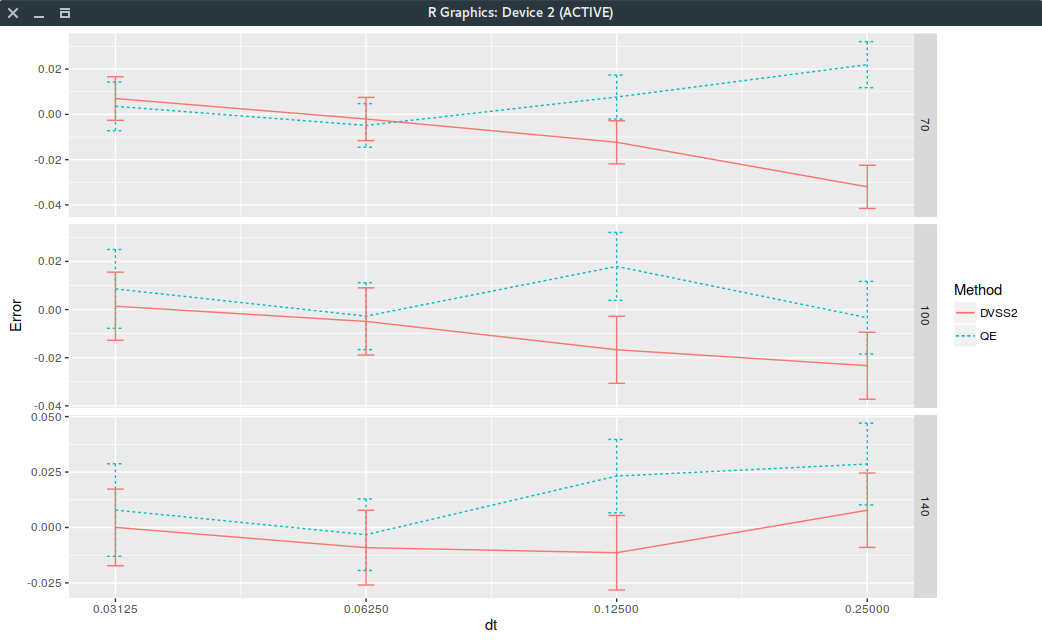Error on Leif Andersen Case III.

Update January 23rd The scheme does not behave very well on Vanilla forward start options.

# Andreasen-Huge interpolation - Don't stay flat

Jesper Andreasen and Brian Huge propose an arbitrage-free interpolation method based on a single-step forward Dupire PDE solution in their paper Volatility interpolation. To do so, they consider a piecewise constant representation of the local volatility in maturity time and strike where the number of constants matches the number of market option prices.

An interesting example that shows some limits to the technique as described in Jesper Andreasen and Brian Huge paper comes from Nabil Kahale paper on an arbitrage-free interpolation of volatilities.option volatilities for the SPX500 in October 1995.

Yes, the data is quite old, and as a result, not of so great quality. But it will well illustrate the issue. The calibration of the piecewise constant volatilities on a uniform grid of 200 points (on the log-transformed problem) leads to a perfect fit: the market vols are exactly reproduced by the following piecewise constant vols: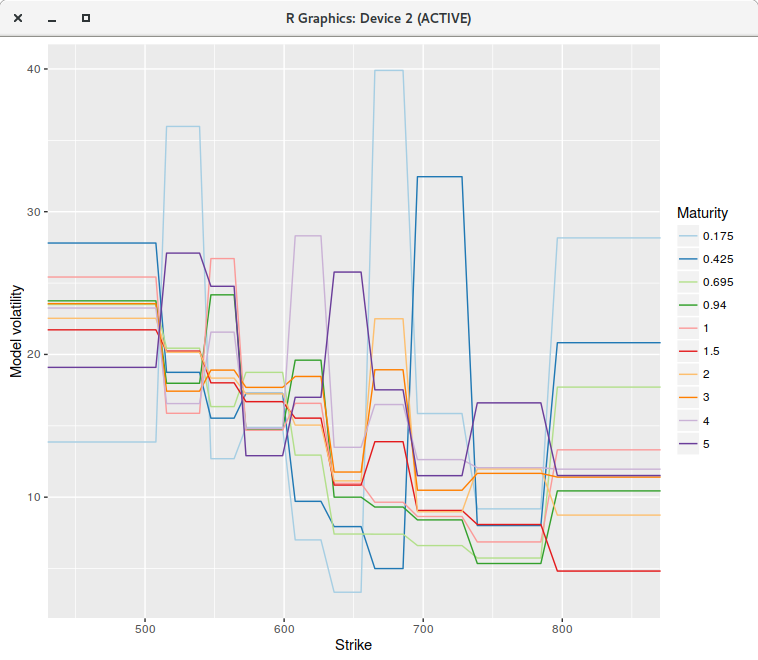piecewise constant model on a grid of 200 points.

However, if we increase the number of points to 400 or even much more (to 2000 for example), the fit is not perfect anymore, and some of the piecewise constant vols explode (for the first two maturities), even though there is no arbitrage in the market option prices.piecewise constant model on a grid of 400 points.

The single step continuous model can not represent the market implied volatilities, while for some reason, the discrete model with 200 points can. Note that the model vols were capped, otherwise they would explode even higher.

If instead of using a piecewise constant representation, we consider a continuous piecewise linear interpolation (a linear spline with flat extrapolation), where each node falls on the grid point closest market strike, the calibration becomes stable regardless of the number of grid points.piecewise linear model on a grid of 400 points.

The RMSE is back to be close to machine epsilon. As a side effect the Levenberg-Marquardt minimization takes much less iterations to converge, either with 200 or 400 points when compared to the piecewise constant model, likely because the objective function derivatives are smoother. In the most favorable case for the piecewise constant model, the minimization with the linear model requires about 40\% less iterations.

We could also interpolate with a cubic spline, as long as we make sure that the volatility does not go below zero, for example by imposing a limit on the derivative values.

Overall, this raises questions on the interest of the numerically much more complex continuous time version of the piecewise-constant model as described in Filling the gaps by Alex Lipton and Artur Sepp: a piecewise constant representation is too restrictive.

# Put-Call parity and the log-transformed Black-Scholes PDE

We will assume zero interest rates and no dividends on the asset $$S$$ for clarity. The results can be easily generalized to the case with non-zero interest rates and dividends. Under those assumptions, the Black-Scholes PDE is: $$\frac{\partial V}{\partial t} + \frac{1}{2}\sigma^2 S^2 \frac{\partial^2 V}{\partial S^2} = 0.$$

An implicit Euler discretisation on a uniform grid in $$S$$ of width $$h$$ with linear boundary conditions (zero Gamma) leads to:

$$V^{k+1}_i - V^{k}_i = \frac{1}{2}\sigma^2 \Delta t S_i^2 \frac{V^{k}_{i+1}-2V^k_{i}+V^{k}_{i-1}}{h^2}.$$ for $$i=1,…,m-1$$ with boundaries $$V^{k+1}_i - V^{k}_i = 0.$$ for $$i=0,m$$.

This forms a linear system $$M \cdot V^k = V^{k+1}$$ with $$M$$ is a tridiagonal matrix where each of its rows sums to 1 exactly. Furthermore, the payoff corresponding to the forward price $$V_i = S_i$$ is exactly preserved as well by such a system as the discretized second derivative will be exactly zero. The scheme can be seen as preserving the zero-th and first moments.

As a consequence, by linearity, the put-call parity relationship will hold exactly (note that in between nodes, any interpolation used should also be consistent with the put-call parity for the result to be more general).

This result stays true for a non-uniform discretisation, and with other finite difference schemes as shown in this paper.

It is common to consider the log-transformed problem in $$X = \ln(S)$$ as the diffusion is constant then, and a uniform grid much more adapted to the process. $$\frac{\partial V}{\partial t} + \frac{1}{2}\sigma^2 \frac{\partial^2 V}{\partial X^2}-\frac{1}{2}\sigma^2 \frac{\partial V}{\partial X} = 0.$$

An implicit Euler discretisation on a uniform grid in $$X$$ of width $$h$$ with linear boundary conditions (zero Gamma in $$S$$ ) leads to:

$$V^{k+1}_i - V^{k}_i = \frac{1}{2}\sigma^2 \Delta t \frac{V^{k}_{i+1}-2V^k_{i}+V^{k}_{i-1}}{h^2}-\frac{1}{2}\sigma^2 \Delta t \frac{V^{k}_{i+1}-V^{k}_{i-1}}{2h}.$$ for $$i=1,…,m-1$$ with boundaries $$V^{k+1}_i - V^{k}_i = 0.$$ for $$i=0,m$$.

Such a scheme will not preserve the forward price anymore. This is because now, the forward price is $$V_i = e^{X_i}$$. In particular, it is not linear in $$X$$.

It is possible to preserve the forward by changing slightly the diffusion coefficient, very much as in the exponential fitting idea. The difference is that, here, we are not interested in handling a large drift (when compared to the diffusion) without oscillations, but merely to preserve the forward exactly. We want the adjusted volatility $$\bar{\sigma}$$ to solve $$\frac{1}{2}\bar{\sigma}^2 \frac{e^{h}-2+e^{-h}}{h^2}-\frac{1}{2}\sigma^2 \frac{e^{h}-e^{-h}}{2h}=0.$$ Note that the discretised drift does not change, only the discretised diffusion term. The solution is: $$\bar{\sigma}^2 = \frac{\sigma^2 h}{2} \coth\left(\frac{h}{2} \right) .$$ This needs to be applied only for $$i=1,…,m-1$$.

This is actually the same adjustment as the exponential fitting technique with a drift of zero. For a non-zero drift, the two adjustments would differ, as the exact forward adjustment will stay the same, along with an adjusted discrete drift.

# Benaim et al. extrapolation does not work on equities

We have seen earlier that a simple parabola allows to capture the smile of AAPL 1m options surprisingly well. For very high and very low strikes, the parabola does not obey Lee’s moments formula (the behavior in the wings needs to be at most linear in variance/log-moneyness).

Extrapolating the volatility smile in the low or high strikes in a smooth $$C^2$$ fashion is however not easy. A surprisingly popular so called “arbitrage-free” method is the extrapolation of Benaim, Dodgson and Kainth developed to remedy the negative density of SABR in interest rates as well as to give more control over the wings.

The call options prices (right wing) are extrapolated as: $$C(K) = \frac{1}{K^\nu} e^{a_R + \frac{b_R}{K} + \frac{c_R}{K^2}} \text{.}$$ $$C^2$$ continuity conditions for the right wing at strike $$K_R$$ lead to: $$c_R =\frac{{C’}_R}{C_R}K_{R}^3+ \frac{1}{2}K_{R}^2 \left(K_{R}^2 \left(- \frac{{C’}_{R}^{2}}{C_{R}^2}+ \frac{{C”}_R}{C_R}\right) + \nu \right)\text{,}$$ $$b_R = - \frac{{C’}_R}{C_R} K_R^2 - \nu K_R-2 \frac{c_R}{K_R}\text{,}$$ $$a_R = \log(C_R)+ \nu \log(K_R) - \frac{b_R}{K_R} - \frac{c_R}{K_{R}^2}\text{.}$$ The $$\ nu$$ parameters allow to adjust the shape of the extrapolation.

Unfortunately it does not really work for equities. Very often, the extrapolation will explode, which is what we wanted to avoid in the first place. We illustrate it here on our best fit parabola of the AAPL 1m options: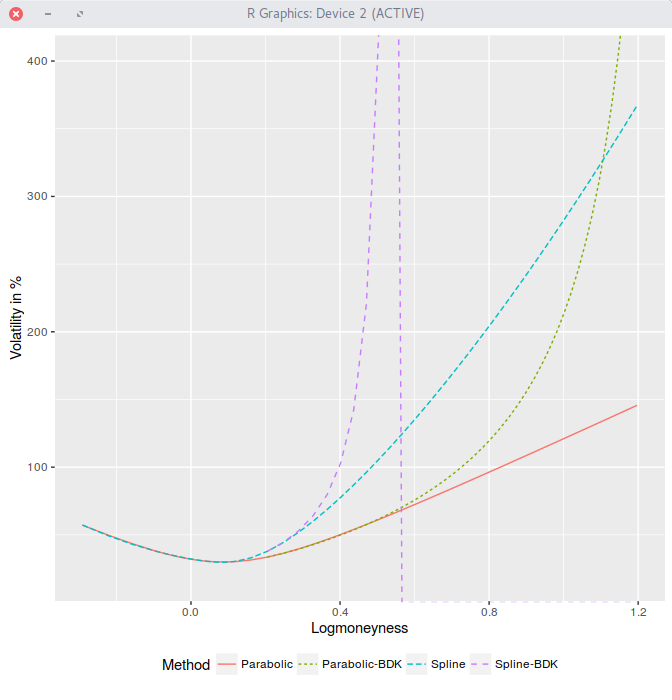BDK explodes on AAPL 1m options.

The $$\nu$$ parameter does not help either.

Update Dec 5 2016 Here are details about call option price, slope, curvature: The strike cutoff is $$K_R=115.00001307390328$$. For the parabola,

\begin{align} C(K_R)&=0.014516747104643029,\ C’(K_R)&=-0.002899391065224203,\ C”(K_R)&=0.000750774042345718. \end{align}

And the BDK parameters for the right wing:

$$a_R=34.279812, b_R=-10292.881677, c_R=737108.461636.$$

For the least squares spline, \begin{align} C(K_R)&=0.03892674300426042,\ C’(K_R)&=-0.00171386452499034,\ C”(K_R)&=0.0007835686926501496. \end{align} which results in $$a_R=131.894286, b_R=-26839.217814, c_R=1550285.706087.$$

Submit to reddit
 © 2006-16 FabienThis work is licensed under a Creative Commons Attribution 4.0 International License.